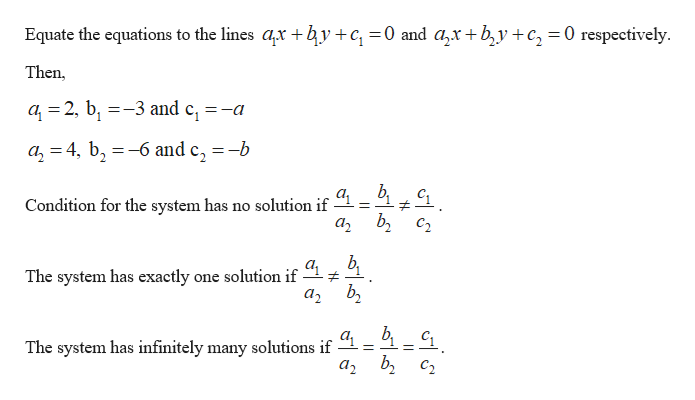Under what conditions on a and b will the following linear system have no solutions, one solution, infinitely many solu- tions?2x − 3y = a4x − 6y = b

Question

Under what conditions on a and b will the following linear system have no solutions, one solution, infinitely many solu- tions?

2x − 3y = a

4x − 6y = b

Step 1

The given linear equations are,

2x – 3y = a and 4...help_outlineImage TranscriptioncloseEquate the equations to the lines ar by+c =0 and azx+b,y+c, = 0 respectively - Then, a 2, b3 and e, =-a 1 a24, b6 and c, =-b Condition for the system has no solution if # C2 The system has exactly one solution if The system has infinitely many solutions if "1= 1-1 fullscreen

Want to see the full answer?

See Solution

Want to see this answer and more?

Our solutions are written by experts, many with advanced degrees, and available 24/7

See Solution
Tagged in

Math